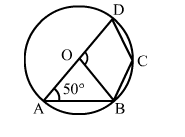# In the given figure, O is the centre of a circle and ∠OAB = 50°.

Question:

In the given figure, O is the centre of a circle and OAB = 50°. Then, ∠BOD = ?
(a) 130°

(b) 50°
(c) 100°
(d) 80°Solution:

(c) 100°
OA = OB  (Radii of a circle)
⇒ ∠OBA = ∠OAB = 50°
In
Δ OAB, we have:
∠ OAB + ∠OBA + ∠AOB = 180°    (Angle sum property of a triangle)
⇒ 50° + 50° + ∠AOB = 180°
⇒ ∠AOB = (180° - 100°) = 80°
Since ∠AOB + ∠BOD = 180°
(Linear pair)
∴ ∠BOD = (180° - 80°) = 100°